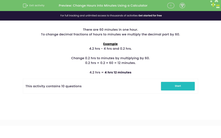# Change Hours into Minutes Using a Calculator

In this worksheet, students will use a calculator to change hours into minutes.Key stage:  KS 3

Curriculum topic:   Number

Curriculum subtopic:   Use Calculators/Technology for Accuracy

Difficulty level:#### Worksheet Overview

There are 60 minutes in one hour.

To change decimal fractions of hours to minutes, we multiply the decimal part by 60.Example

4.2 hrs is 4 hrs and 0.2 hrs.

Change 0.2 hrs to minutes by multiplying by 60.

0.2 hrs = 0.2 × 60 = 12 minutes.

4.2 hrs = 4 hrs 12 minutes

Does that make sense?

Let's try some questions together now.

### What is EdPlace?

We're your National Curriculum aligned online education content provider helping each child succeed in English, maths and science from year 1 to GCSE. With an EdPlace account you’ll be able to track and measure progress, helping each child achieve their best. We build confidence and attainment by personalising each child’s learning at a level that suits them.

Get started